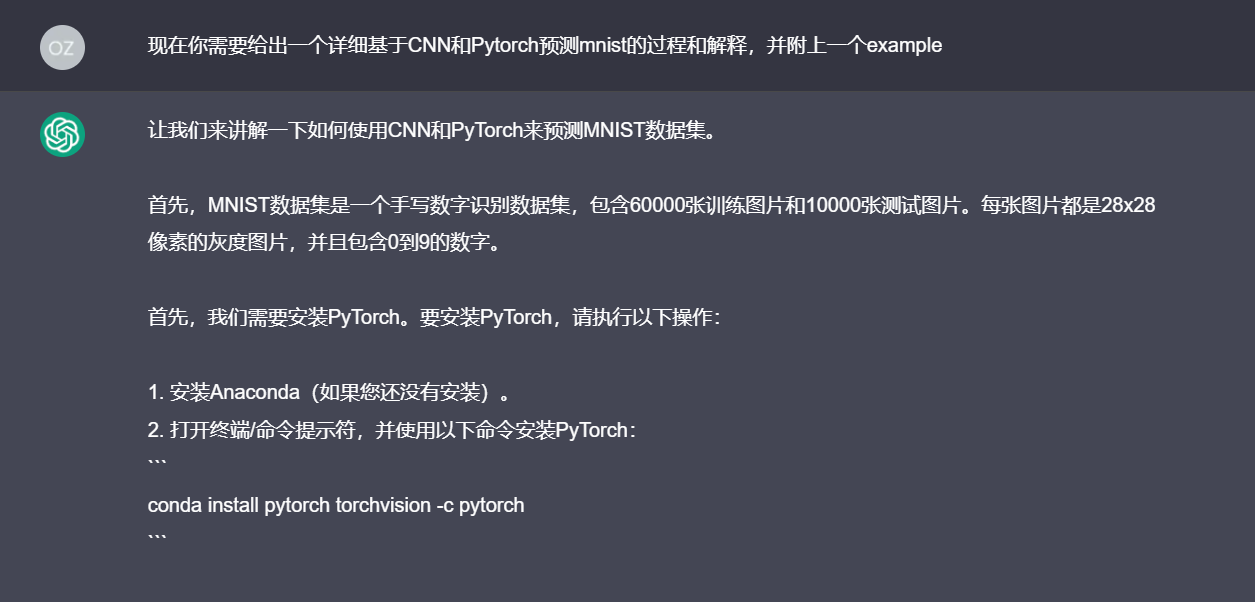Zeeland

The mixture of software dev+Iot+ml+anything

• 累计撰写 57 篇文章
• 累计创建 43 个标签
• 累计收到 7 条评论

### 目 录CONTENT# 当我用ChatGPT中学习CNN卷积神经网络2022-12-10 / 0 评论 / 0 点赞 / 237 阅读 / 1,757 字

## Introduction

ChatGPT大火，在这一段时间并没有觉得ChatGPT特别厉害，最多就是一个基于生成式对话的NLP模型罢了，直到我看到了AI扮演Linux虚拟机，能管理文件&编程&开浏览器，还能跟自己「套娃」聊天 | ChatGPT新玩法，于是好奇的我打算去讨教一下ChatGPT，于是就有了以下的场面。## 我和ChatGPT的对话

Q: 现在你需要给出一个详细基于CNN和Pytorch预测mnist的过程和解释，并附上一个example

ChatGPT:

1. 安装Anaconda（如果您还没有安装）。
2. 打开终端/命令提示符，并使用以下命令安装PyTorch：
conda install pytorch torchvision -c pytorch


import torch
import torchvision
import torch.nn as nn
import torch.nn.functional as F
import torch.optim as optim


train_dataset = torchvision.datasets.MNIST(root='./data', train=True, transform=torchvision.transforms.ToTensor(), download=True)


Q: 继续

ChatGPT:

train_loader = torch.utils.data.DataLoader(dataset=train_dataset, batch_size=64, shuffle=True)


class CNN(nn.Module):
def __init__(self):
super(CNN, self).__init__()
self.conv1 = nn.Conv2d(in_channels=1, out_channels=16, kernel_size=5, stride=1, padding=2)
self.pool = nn.MaxPool2d(kernel_size=2, stride=2)
self.conv2 = nn.Conv2d(in_channels=16, out_channels=32, kernel_size=5, stride=1, padding=2)
self.fc1 = nn.Linear(32 * 7 * 7, 10)

def forward(self, x):
x = self.pool(F.relu(self.conv1(x)))
x = self.pool(F.relu(self.conv2(x)))
x = x.view(x.size(0), -1)
x = self.fc1(x)
return x


Q: 继续

ChatGPT:

criterion = nn.CrossEntropyLoss()


optimizer = optim.SGD(model.parameters(), lr=0.01)


1. 将数据输入网络，并使用前向传播函数计算预测值。
2. 计算损失函数的值。
3. 计算损失函数的梯度。
4. 使用优化器更新网络参数。

# 训练网络
for epoch in range(5):
for batch_idx, (data, targets) in enumerate(train_loader):
# 计算预测值
outputs = model(data)
# 计算损失函数
loss = criterion(outputs, targets)
# 梯度清零
# 反向传播
loss.backward()
# 更新参数
optimizer.step()


Q： 继续

ChatGPT:

1. 将数据输入网络，并使用前向传播函数计算预测值。
2. 计算损失函数的值。
3. 计算精度。

# 评估网络
model.eval()
correct = 0
total = 0
outputs = model(data)
_, predicted = torch.max(outputs.data, 1)
total += targets.size(0)
correct += (predicted == targets).sum().item()
print('精度：{}%'.format(100 * correct / total))


0